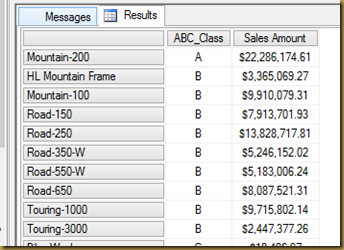## Friday, August 29, 2014

### ABC Classification

I was asked to make a simple ABC classification for a sales measures. I combined exists with TopPercent to identify:

• Class A = between the top 0 and 20 %
• Class B = between the top 20 and 80%
• Class C = between the top 80 and 100%

with
member [Measures].[ABC_Class] AS
CASE
/* A Catorgie = first 20% */
WHEN (
NOT isempty(
(exists (
[Product].[Model Name].currentmember,
/* Get the top 20 percent */
{TOPPERCENT([Product].[Product Model Lines].[Model].Members, 20,[Measures].[Sales Amount])}
).item(0),
[Measures].[Sales Amount])
) ) THEN "A" /* Null if not A */
/* B Catogorie = between 20 and 80 % */
WHEN (
NOT isempty(
(exists (
[Product].[Model Name].currentmember,
/* Get the top 80 percent */
{TOPPERCENT([Product].[Product Model Lines].[Model].Members, 80,[Measures].[Sales Amount])}
).item(0),
[Measures].[Sales Amount])
) ) THEN "B" /* Null if not A or B */
/* C Catogorie > 80% */
WHEN (
NOT isempty(
(exists (
[Product].[Model Name].currentmember,
/* Get the top 100 percent */
{TOPPERCENT([Product].[Product Model Lines].[Model].Members, 100,[Measures].[Sales Amount])}
).item(0),
[Measures].[Sales Amount])
) ) THEN "C"
END

select {
[Measures].[ABC_Class], [Measures].[Sales Amount]
} on Columns ,
NonEmpty(ORDER([Product].[Model Name].Children,[Measures].[ABC_Class]) ) on Rows
from